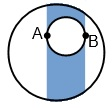BrainDen.com - Brain Teasers
• 0

# Obtuse triangles in a circle

## Recommended Posts

• 0
On 2/12/2018 at 2:36 AM, bonanova said:

One might start by constraining the points to be on the circle's perimeter.

Spoiler

...nevermind

##### Share on other sites
• 0

Not an answer, but a description of a potential approach and what would still be needed to make it work since this has gone for a while without being cracked.

Spoiler

If you randomly place two points A and B in the circle:

Draw the line segment connecting those two points, then draw a stripe covering and perpendicular to that line segment. If the third point C is outside of that stripe, then the angle at either A or B will be obtuse. Also, cut out the circle with diameter from A to B from the stripe, because if point C is within that circle then the angle at C is obtuse. If point C is within what’s left of the stripe, then the triangle is acute (or right).To figure the area of that stripe: Rotate the circle so the stripe is going vertically. Then measure the distance from the left edge of the stripe to the left edge of the circle, and from the right edge of the stripe to the right edge of the circle. The area that’s cut off from the stripe on either side can be calculated by taking the area of the circle’s wedge including that stuff that’s cut off, and subtracting the area of the isosceles triangle from the center’s origin that goes out to that area that’s cut off, as shown in the figure.After calculating the area of those two edges that get cut off, you can calculate the area of the circle with diameter A to B, and then subtract all of those areas from the total area of the circle to get the area of the stripe.

That calculation would depend only on the x coordinates of points A and B after you rotate the circle to make line A-B parallel to the x-axis. If you can calculate the expected distribution of those two points’ x coordinates then the problem can be solved with those calculations. But describing that distribution given random placement of those two points has proven more difficult that I would’ve expected.

## Join the conversation

You can post now and register later. If you have an account, sign in now to post with your account.×   Pasted as rich text.   Paste as plain text instead

Only 75 emoji are allowed.

×   Your previous content has been restored.   Clear editor

×   You cannot paste images directly. Upload or insert images from URL.